### Examples - Electromagnetism¶

Last edited: March 22nd 2018

### Introduction¶

In many applications in physics, chemistry and biology, it is crucial to determine which atoms and molecules are created in different processes in experiments. Among the techniques that can do this is mass spectrometry. The physical principle behind the technique is quite simple and based on Newtons second law $\vec F = m\vec a$. This shows that particles with different mass achieves different acceleration if they are exposed to the same force. Electric or magnetic forces are often used. In a mass spectrometer a number of atoms or molecules are ionized and then sorted based on their mass to charge ratio. In this notebook we discuss a so-called quadrupole mass spectrometer.

### Setup¶

A quadrupole mass spectrometer consist of four parallel (and often sylindrical) electrodes of length $L$ in each corner of a square, as seen in Figure 1 below.Figure 1: Sketch of the quadrupole mass spectrometer. The figure is acquired from: http://www.bris.ac.uk/nerclsmsf/techniques/gcms.html.

The electrodes are located a distance $r_0$ from the central axis. Ionized particles enter through a narrow slit with a velocity $v$ parallel to the electrodes, also seen in Figure 1 above. The spectrometer has a detector slit at the end of the electrodes, here assumed to be $r_0/4$. The electrodes are pairwise connected to the potential $V(t) = \pm (V_{DC}+V_{AC}\cos\omega t)$. This way two diametrically opposed electrodes have the potential $+V$ while the other two have $-V$. The motion of particles will depend on the amplitudes $|V_{AC}|$ and $|V_{DC}|$ and the angular frequency $\omega$.

The motion of heavy particles are weakly affected by the alternating current (AC) voltage component $V_{AC}$, while the motion of light particles are influenced strongly by this component. Thus, $V_{AC}$ operates as a filter against the particles of low mass to charge ratio, while the direct current (DC) voltage component $V_{DC}$ can be viewed as a filter against the heavier particles. Hence, if we are to choose a good combination of $|V_{AC}|$ and $|V_{DC}|$ only particles with a given mass to charge ratio will have a stable path through the apparatus.

For simplicity, we assume that all the ions have the same charge $q$ such that we determine their mass $m$.

As always, we start by importing packages, setting figure parameters and defining some variables. Let $L=0.1$ m be the length of the electrodes and $\omega = 10^7$ Hz be the frequency of $V_{AC}$. We will also use $r_0=3$ mm and further assume that the speed of incoming particles is $v=3000$ m/s. The time it takes a particle to move through the apparatus is thus $t_{max}=20$ $\mu$s. We will use a coordinate system where $z$ is parallel to the electrodes.

In :
# Import libraries
import numpy as np
import matplotlib.pyplot as plt
from mpl_toolkits.mplot3d import Axes3D

# Parameters
omega = 1e7  # [Hz] Frequency of the AC
v0 = 5000  # [m/s] Speed of the incident ion
L = 0.1  # [m] Length of the apparatus/electrodes
u = 1.66*1e-27  # [m] Atomic unit
q = 1.6022*1e-19  # [C] Elementary charge
r0 = 0.003  # [m] Length from the origin to the electrodes

In :
# Set common figure parameters
newparams = {'font.size': 14, 'figure.figsize': (16, 6),
'mathtext.fontset': 'stix', 'font.family': 'STIXGeneral',
'lines.linewidth': 2}
plt.rcParams.update(newparams)


### The electric and potential fields¶

A cross-section of the quadrupole represents four circular charges in the plane. Gauss' law,

$$\Phi_E = \oint_S\vec E\cdot \text{d}\vec A,$$

tells us that the electric field outside the circular charges is equal to the electric field around a point charge. We can therefore find the electric field and potential, as shown in our notebook titled Electric fields and potentials from point charges. Doing the calculation gives us

$$V(r)\propto \ln \frac{r_1r_2}{r_3r_4},$$

where $r_i$ is the distance to the $i$th electrode. For simplicity, we will be using the hyperbolic potential

$$V(x,y,t)=(V_{DC}+V_{AC}\cos \omega t)\frac{x^2-y^2}{r_0^2},$$

which gives the electric field

$$\vec E(x,y,t)=-\nabla V(x,y,t) = (V_{DC}+V_{AC}\cos \omega t)\frac{2}{r_0^2}(-x,y).$$

Let us plot the shape of the fields, setting $V_{AC} = 0$ and $V_{DC}=1$. Note that the approximate hyperbolic potential is qualitatively similar to the true potential.

In :
resolution = 200j
equipotential_curves = 30

y, x = np.mgrid[-2*r0:2*r0:resolution, -2*r0:2*r0:resolution]

# Calculating the hyperbolic potential and electric field
Vhyp = (x**2 - y**2)/r0**2
Exhyp = -2/r0**2*x
Eyhyp = 2/r0**2*y

# Calculating the potential and electric field of the point charges
r1 = np.sqrt((x - r0)**2 + y**2)
r2 = np.sqrt((x + r0)**2 + y**2)
r3 = np.sqrt(x**2 + (y - r0)**2)
r4 = np.sqrt(x**2 + (y + r0)**2)
Vlin = -np.log(r1*r2/(r3*r4))

plt.figure()
# Plot the hyperbolic potential and electric field
plt.subplot(121)
plt.streamplot(x/r0, y/r0, Exhyp, Eyhyp)
plt.contour(x/r0, y/r0, Vhyp, equipotential_curves)
plt.plot([-1, 1], [0, 0], 'ro')
plt.plot([0, 0], [-1, 1], 'bo')
plt.title('Hyperbolic potential and electric field')

# Plot the potential and electric field of the point charges
plt.subplot(122)
plt.streamplot(x/r0, y/r0, Exlin, Eylin)
plt.contour(x/r0, y/r0, Vlin, equipotential_curves)
plt.plot([-1, 1], [0, 0], 'ro')
plt.plot([0, 0], [-1, 1], 'bo')
plt.title('Potential and electric field of the point charges')
plt.show()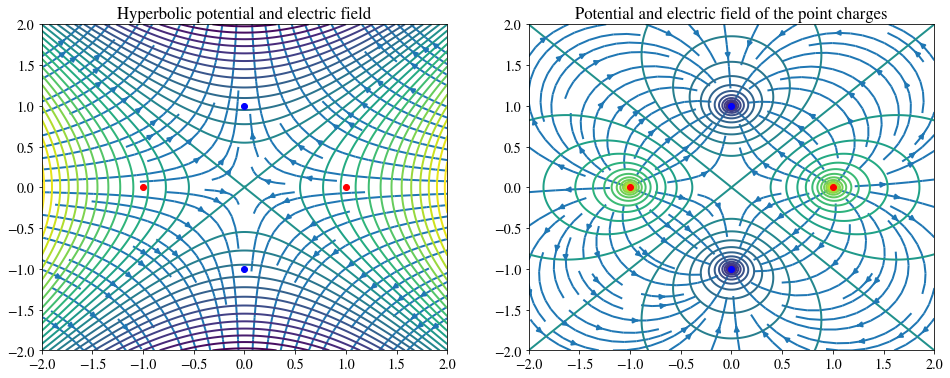### Equation of motion¶

The force $\vec F_E = q\vec E/m$ is excerted on the particle in the electric field. Newton's second law and the equation for the electric field gives the equations of motion

$$\frac{\text{d}v}{\text{d}t} = \frac{2q}{mr_0^2}(V_{DC}+V_{AC}\cos \omega t)(-x,y),$$$$\frac{\text{d}\vec x}{\text{d}t}=\vec v.$$

These equations can be solved e.g. using one of the methods described in one of our Ordinary Differential Equations modules, used in the examples Planetary Motion - Three Body Problem or Planetary Motion - One Body Problem. Here we use the 4th order Runge-Kutta method, trading off computational speed for simplicity.

In :
def rk4_step(X, t, dt, m, VDC, VAC):
""" Performs one step of the fourth order Runge-Kutta (RK4) method.
:X:   numpy-array, [x, y, vx, vy].
:x:  float. x-position.
:y: float. y-direction.
:vx: float. Velocity in x-direction.
:vy: float. Velocity in y-direction.
:t:   float. Time.
:dt:  float. Time step.
:m:   float. Mass of the ion.
:VDC: float. Amplitude of DC-voltage.
:VAC: float. Amplitude of AC-voltage.
:Returns: numpy-array, [x, y, vx, vy]. Position and speed at t+dt.
"""
def f(X, t):
""" Calculates the right hand side of the equations of motion."""
val = 2*q/m*(VDC + VAC*np.cos(omega*t))/r0**2
return np.array([X, X, -val*X, val*X])
s1 = f(X, t)
s2 = f(X + dt/2*s1, t + dt/2)
s3 = f(X + dt/2*s2, t + dt/2)
s4 = f(X + dt*s3, t + dt)
return X + dt/6*(s1 + 2*s2 + 2*s3 + s4)


### Analytical solution and error estimate¶

For the moment we will assume that we are studying an $N_2^+$ ion with mass $m=28u$. Given the start position $(x_0, 0)$ a simple analytical solution is

$$x(t)=x_0\cdot \cos\left(\sqrt{\frac{2qV_{DC}}{mr_0^2}}\cdot t\right).$$

We can use this to estimate an accuracy for our numerical methods and check that they are correct.

Since the analytical solution is known in this special case, we can quantify the error. For our purposes the maximum relative error is a good estimate for the error

$$\text{error}\equiv \frac{\max_{t\in[0,t_\text{max}]}\left|\vec x(t)-\vec x_\text{exact}(t)\right|}{\max_{t\in[0,t_\text{max}]}\left|\vec x_\text{exact}(t)\right|}.$$

Let us plot both the analytical the numerical solutions and estimate the error. We choose $m=28u$, $x_0 = 1$ mm, $V_{DC}=10$.

In :
# Parameters
m = 28*u
VDC = 10
VAC = 0

n = 300  # Iterations
[y0, x0] = [0, 0.001]  # [m] Initial position of particle

tmax = L/v0  # [s] The time when the particle is detected
dt = tmax/(n - 1)  # Step length
t = np.linspace(0, tmax, n, True)  # Time array

X = np.zeros([n, 4])  # Matrix for the position and velocity for each iteration
X[0, :] = [x0, y0, 0, 0]  # Initial conditions for position and velocity

# Iterative method solving the differential equation. See a notebook
for i in range(1, n):
X[i, :] = rk4_step(X[i - 1, :], t[i - 1], dt, m, VDC, VAC)

# Calculate analytical solution and error
analytical_solution = x0*np.cos(np.sqrt(q*2*VDC/(r0**2*m))*t)
error = (np.max(np.abs(X[:, 0] - analytical_solution))/np.max(analytical_solution))

# Plotting the result
plt.figure()
plt.plot(t*1e6, analytical_solution*1e3, label='Analytical')
plt.plot(t*1e6, X[:, 0]*1e3, label='Numerical')
plt.legend()
plt.xlabel('$t$, [$\mu$s]')
plt.ylabel('$x$, [mm]')

print("Error: %.2f%%" % (100*error))

Error: 0.05%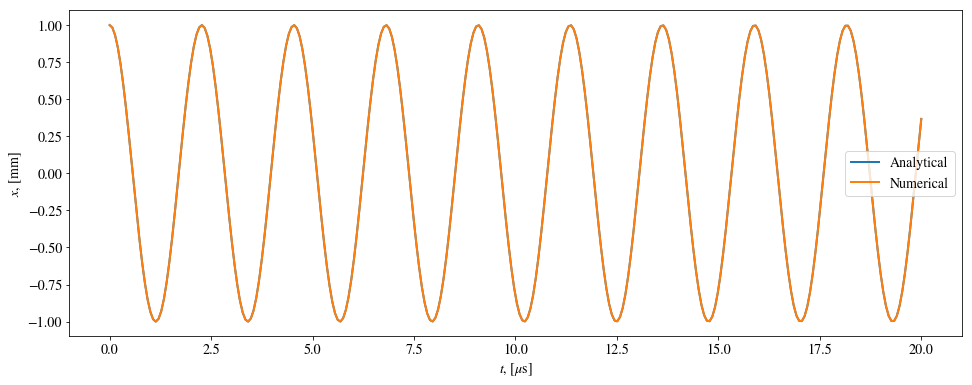Note that large accelerations (and thus large $V_{AC}$ and $V_{DC}$) leads to higher error. As a compromise between computational speed and accuracy, we will choose $n=300$ steps from now on.

### Arbitrary start position and voltages¶

Using everything we have learned so far, it is easy to compute the trajectory of a particle with an arbitrary start position (within the apparatus) and different voltages. If the particle hits one of the electrodes ($r^2=x^2+y^2$ < $r_0^2$) we can termitate the running loop and conclude that the particle was not detected. If the position at $t_\text{max}$ is inside the detector ($r^2$ < $r_0^2/4$) the particle is detected. We start by using $x_0=y_0 = 1$ mm, $V_{AC}=45$ and $V_{DC}=5$ (test other parameters for yourself!).

In :
# Parameters
[x0, y0] = [0.001, 0.001]
VAC = 45
VDC = 5
n = 300

# Performing the calculations as earlier
dt = tmax/(n - 1)
t = np.linspace(0, tmax, n, True)
X = np.zeros([n, 4])
X[0, :] = [x0, y0, 0, 0]

for i in range(1, n):
X[i, :] = rk4_step(X[i - 1, :], t[i - 1], dt, m, VDC, VAC)
if X[i, 0]**2 + X[i, 1]**2 >= r0**2:
print('The particle collided with one of the electrodes.\n')
break
if (X[n - 1, 0]**2 + X[n - 1, 1]**2 <= (r0**2)/4) & (i == n):
print('The particle was detected.\n')
elif i == n:
print('The particle was not detected.\n')

# 2D plot of the trajectory in the xz and zy plane
plt.figure()
plt.plot(t[0:i]/tmax*L, X[0:i, 0], label='$x$ position')
plt.plot(t[0:i]/tmax*L, X[0:i, 1], label='$y$ position')
plt.xlabel('t, [$\mu$s]')
plt.ylabel('[mm]')
plt.title('Trajectory using $x_0=$%.2f mm, $x_0=$%.2f mm, $V_{AC}$=%d V and $V_{AC}$=%d V.'
% (x0*1e3, y0*1e3, VAC, VDC))
plt.legend();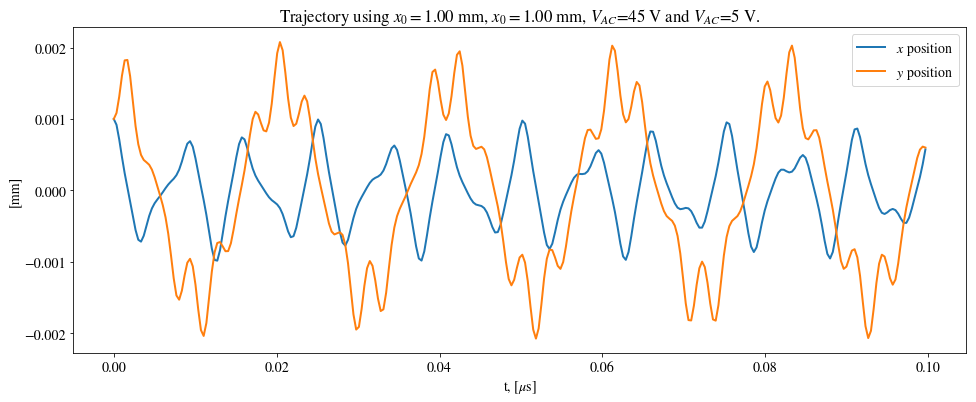### Stability diagram¶

It is instructive to check for which voltages $V_{AC}$ and $V_{DC}$ the particle with a specific mass passes through the detector. If we know this, we can simply change the voltages such that only ions with a given mass to charge ratio are detected. To do this we start by creating a function which compute the trajectory and returns whether or not the particle hit the electrodes.

In :
def collision_test(X,VDC,VAC,m):
"""Calculates whether a particle collides with the electrodes
with given parameters.
:X:   numpy-array, [x0, y0, vx0, vy0].
:x0:  float. Initial x-position.
:y0:  float. Initial y-direction.
:vx0: float. Initial velocity in x-direction.
:vy0: float. Initial velocity in y-direction.
:m:   float. Mass of the ion.
:VDC: float. Amplitude of DC-voltage.
:VAC: float. Amplitude of AC-voltage.
:Returns: bool. Collision and detection of the particle.
"""
t = 0
for k in range(1, n):
X = rk4_step(X, t, dt, m, VDC, VAC)
t = t + dt
if X**2 + X**2 >= r0**2:
break
r = X**2 + X**2
return (r <= r0**2, r<=r0**2/4)


We now iterate through a given set of voltages, say $0 \text{V}\leq V_{AC}\leq 60 \text{V}$ and $1\text{V}\leq V_{DC}\leq 10\text{V}$ with the same initial conditions as earlier, but choosing the start position close to the origin.

We iterate through $V_{AC}$ from 0. From the beginning the particle will not hit the electrodes. But after increasing the voltage to a particular value the particle will always collide... Why? Try for yourself! We can therefore terminate the $V_{AC}$ loop after we encounter this first collision.

The below diagram will be discussed in more detail after it is plotted.

In :
m = 28*u
[x0, y0] = [0.0001, 0.0001]

X0 = [x0, y0, 0, 0]

[VAC_min, VAC_max] = [10, 60]
[VDC_min, VDC_max] = [1, 9]
VDC_step = 0.1
VAC_step = 0.1
counter = 0

# Creating a figure and setting figure labels
fig = plt.figure()
plt.axis([VAC_min, VAC_max, VDC_min, VDC_max])
plt.xlabel(r'$V_{AC}$, [V]')
plt.ylabel(r'$V_{DC}$, [V]')
plt.title('Stability diagram for $m = %du$' % (m/u))

stability_region = np.zeros([2, int((VAC_max - VAC_min)/VAC_step)]);
V_AC = np.arange(VAC_min, VAC_max, VAC_step)
V_DC = np.arange(VDC_min, VDC_max, VDC_step)
for i in range(0, len(V_AC)):
for j in range(0, len(V_DC)):
collision, detection = collision_test(X0, V_DC[j], V_AC[i], m)
if (not collision):  # Check if the particle hit the detector
stability_region[:, counter] = [V_AC[i], V_DC[j]];
counter = counter + 1;
break
#    plt.plot(V_AC[i], V_DC[j], 'r.');
#else:
#    break
# To plot every single point where the particle does not
# collide with the electrode, simply exchange the three
# non-commented lines above with the three commented ones,
# and comment out the the stability region plot below.

plt.plot(stability_region[0, :], stability_region[1, :])

# Find the peak of the diagram
peak_index = np.argwhere(stability_region[1, :].max() == stability_region[1, :])
VDC_peak = stability_region[1, peak_index]
VAC_peak = stability_region[0, peak_index]
print('\rPeak: V_AC = %.1fV, V_DC = %.1fV\n' %(VDC_peak, VAC_peak))

Peak: V_AC = 7.8V, V_DC = 46.0V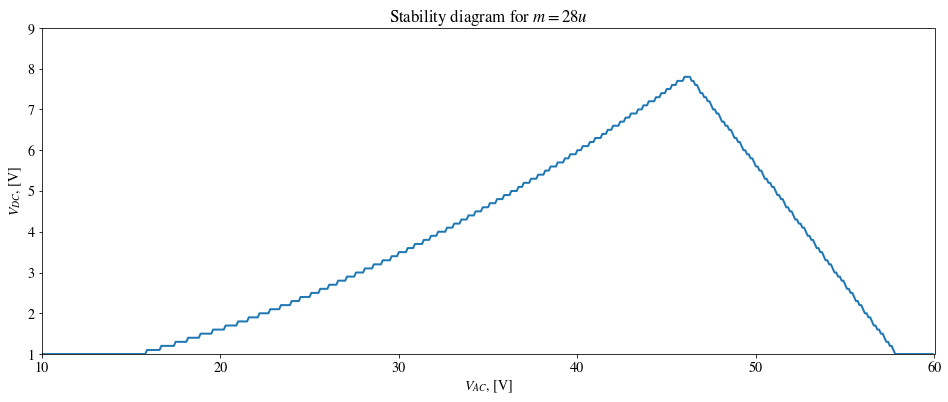All combinations of $V_{AC}$ and $V_{DC}$ below the curve correspond to stable trajectories. Close to the peak at $V_{AC}=46.0$ V and $V_{DC}=7.8$ V only small variations in the mass to charge ratio will determine if the particle if detected or not. In our case we may therefore choose, say $V_{AC}=46.0$ V and $V_{DC}=7.7$ V if we only want to detect the $N_2^+$ ion.

If the amplitudes are well below the curve we have a large uncertainty in which particles are detected. In other words, there may be many particles with different mass to charge ratio that are detected.

The position of the peak for a given particle will depend on its mass. Two particles with different masses, but otherwise the same parameters and inital conditions, will follow the same equations of motion for stable trajectories. Thus, we will have the relations

$$\frac{m_2}{m_1}=\frac{V_{AC,1}}{V_{AC,2}}=\frac{V_{DC,1}}{V_{DC,2}},$$

which can be used to find the peaks for other particles. From this we can see that a peak for a given particle has to lie off the straight line passing through the origin and the peak to a arbitrary particle. In other words, the peaks are collinear.

### A set of particles with random velocities¶

In reality the incident particles will have different start velocity, position and direction. We will now consider a large number of particles, say a 1000, with different start velocity, position and direction and check how many particles have stable trajectories. Let the start position be uniformly distributed on a circular disc with the same radius as the detector ($r_0/2$), the initial speed be $v=5000$ m/s and the initial direction be uniformly distributed over a solid angle of $0.035$ sr (the steradian or square radian is the SI unit of solid angle). Let us do this for the masses in the range $[25u, 33u]$.

With this setup the stability will be more statistically determined. If we choose the voltage amplitudes too close to the peak, only a small amount of the particles will be detected, but they will have the right mass. If we choose the amplitudes well within the stable area, many particles will be detected in a large mass range. We should therefore choose the voltage amplitudes close, but not too close, to the peak. We will be using $V_{AC}=45$ V and $V_{DC}=7$ V. This may be a bit too far within the stable region, but visualizes the discussions above.

In :
VAC = 45
VDC = 7

atomic_masses = np.arange(25, 35)
N = 1000  # Number of particles
D = []  # Holds the percentage of detection

# Performs the calculations as earlier for different masses
for m in atomic_masses*u:
# Distribution in start position
r = r0*np.sqrt(np.random.random(N))
alpha = 2*np.pi*np.random.random(N)
x0 = r*np.cos(alpha)
y0 = r*np.sin(alpha)

# Distribution of the direction around the z axis
theta = np.random.random(N)*spread  # Angle on the z axis
v0z = v0*np.cos(theta)  # Velocity in z direction
phi = np.random.random(N)*2*np.pi  # Angle in the xy plane
v0x = v0*np.sin(theta)*np.cos(phi)  # Velocity in x direction
v0y = v0*np.sin(theta)*np.sin(phi)  # Velocity in y direction

# Calculates whether the particles with the different initial
# conditions are detected
num_detected = 0
for j in range(0, N):
X = [x0[j], y0[j], v0x[j], v0y[j]]
collision, detection = collision_test(X, VDC, VAC, m)
num_detected += detection
D = np.append(D, num_detected/N*100)

# Plot the result
plt.figure()
plt.bar(atomic_masses - 0.4, D, width=0.8)
plt.ylim((0, 100))
plt.title('Detected particles with random start conditions.')
plt.xlabel('m [$u$]')
plt.ylabel('Detected particles [%]');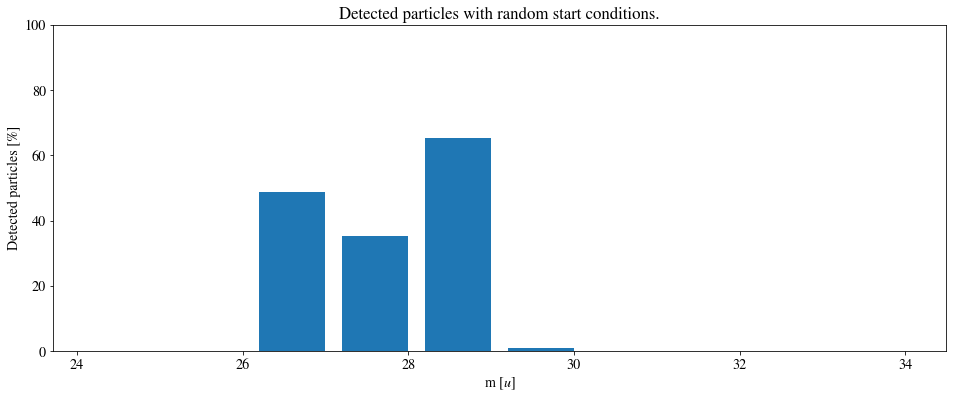### Further questions¶

• What happens to the stability diagram if the start position is not chosen to be close to the origin (e.g. $x_0=y_0=1$mm)? Tips: plot every single point where the trajectory is stable.
• What happens if we plot the detection instead of collisions in the stability diagram?
• Can you explain the events above?

### Resources¶

This notebook is based on an assignment given in the course TMA4320 Introduksjon til vitenskapelige beregninger at NTNU. The assignment was prepared by Ursula Gibson, Vegard Flovik, Jon Andreas Støvneng, Trygve Sørgård and Grunde Wesenberg. The code is based on the answers by Gjert Magne Knutsen, Daniel Halvorsen and Jonas Tjemsland.

Project description in Norwegian, https://wiki.math.ntnu.no/_media/tma4320/2016v/kvadrupol.pdf, acquired: 2016-10-21.